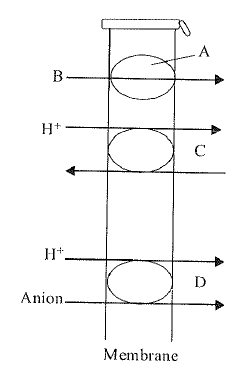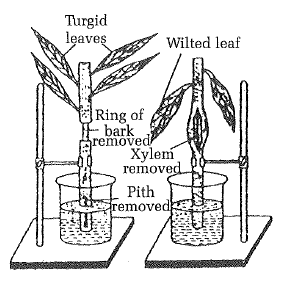What do A, B, C and D represent in the following figure?(a) A : carrier protein, B : symport, C : uniport, D : antiport

(b) A : carrier protein, B : uniport, C : antiport, D : symport

(c) A : carrier protein, B : antiport, C : symport, D : uniport

(d) A : carrier protein, B : uniport, C : symport, D : antiport

Difficulty Level:

What is correct about the movement of substance across the membrane in facilitated diffusion?

(a) It is an active transport.

(b) It cause transport of molecules from low concentration to high concentration.

(c) It is insensitive to inhibitors.

(d) It is a very specific transport.

High Yielding Test Series + Question Bank - NEET 2020

Difficulty Level:

In active transport, carrier protiens are used, which use energy in the form of ATP, to

(a) transport molecules against concentration gradient of cell wall.

(b) transport molecules along concentration gradient of cell membrane.

(c) transport molecules against concentration gradient of cell membrane.

(d) transport molecules along concentration gradient of cell wall.

High Yielding Test Series + Question Bank - NEET 2020

Difficulty Level:

The water logging capacity of garden soil will be determined by measuring the weight of

(a) soil by adding water and humus

(b) water saturated soil then heating the soil till it is dry and then measuring the weight of soil

(c) soil by adding water, boimass and humus

(d) soil from different locations with biomass.

High Yielding Test Series + Question Bank - NEET 2020

Difficulty Level:

The modified equation for water potential is

(a)

(b)

(c) ${\mathrm{\Psi }}_{\mathrm{w}}={\mathrm{\Psi }}_{\mathrm{s}}$

(d) ${\mathrm{\Psi }}_{\mathrm{w}}={\mathrm{\Psi }}_{\mathrm{p}}-{\mathrm{\Psi }}_{\mathrm{s}}$

High Yielding Test Series + Question Bank - NEET 2020

Difficulty Level:

The value of osmotic potential of an electrolyte is always

(a) more than non-electrolyte

(b) less than non-electrolyte

(c) same as non-electrolyte

(d) None of these

High Yielding Test Series + Question Bank - NEET 2020

Difficulty Level:

Identify the process taking place in this experiment.(a) Ringing experiment for translocation of sap
(b) Demonstration of root pressure
(c) Eosin test to demonstrate ascent of sap
(d) Demonstration of transpiration

High Yielding Test Series + Question Bank - NEET 2020

Difficulty Level:

Osmosis is a type of

(a) imbibition of solution

(b) diffusion of solvent

(c) evaporation of water

(d) diffusion of solute

High Yielding Test Series + Question Bank - NEET 2020

Difficulty Level:

Which of the following statement is correct?

(a) DPD = OP - WP

(b) DPD = OP + WP

(c) DPD = WP - OP

(d) DPD= TP + OP

High Yielding Test Series + Question Bank - NEET 2020

Difficulty Level: